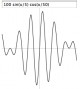Jep 3.3.0 Jep is a Java library for parsing and evaluating mathematical expressions. Commercial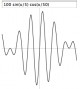Jep Java 3.4.0 Jep is a Java library for parsing and evaluating mathematical expressions. SharewareCalcPad X 1.1 CalcPad X is a "word processor" and evaluator for mathematical expressions! CalcPad X lets you type an expression and evaluate it with a single click. SharewareRapid-Pi 2.1 MS Word add-on that provides a faster way of editing mathematical equations SharewareAcid Library 1.3 Acid Library is a High Performance Math Parser for the .Net Framework. CommercialMaster Math Word Problems 1.2 Master Math Word Problems is an easy to use program to aide students in learning to identify key words that identify mathematical operations and work through mathematical word problems. Shareware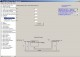ChemMaths 17.4 Software suitable for Engineering,Chemistry/Science, Professionals/education. SharewareMaster Math Word Problems X 1.8.1 Master Math Word Problems is an easy to use program to aid students in learning to identify key words that identify mathematical operations and work through mathematical word problems. Shareware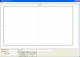BMP EMF GRAPHER 1.1 Code39 Encoder & Fonts empowers you to print Code 39 Barcode Freeware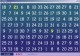Math Worm 2.0 A math puzzle where your worm navigates a maze completing arithmetic equations. Shareware